﻿ 高压油管的压力控制问题研究

# 高压油管的压力控制问题研究On the Problem of Pressure Control of High-pressure Oil Tube

Abstract: The fuel supply system plays an extremely important part in the performance of diesel engine. One mathematical model of dynamical system for the process of fuel entering and ejecting is given in this paper based on the operating principle of high-pressure tubing. Simulation of the operation process of high-pressure oil tube is added to illustrate our results. Compared with the models in the literature, ours is more feasible and useful.

1. 引言

1) 该问题讨论的高压油管其内腔长500 mm，直径10 mm。高压油管左侧有一供油入口A，小孔直径1.4 mm，供油持续的时间长短由单向阀控制，且单向阀每打开一次后需要关闭10 ms。在高压油管右侧有一喷油器每次喷油2.4 ms，工作周期为100 ms，从题目中可以得到喷油器工作时喷油的速率变化图。高压油泵在入口A提供160 MPa的恒定压力，且高压油管内的初始压力为100 MPa。在第一问中，我们需要找到一个合适的单向阀每次的开启时长t，使得高压油管内的压力尽可能稳定在100 MPa左右。而在第二问中，我们需要找到合适的单向阀开启时长使得管内压力分别在经过约2 s、5 s和10 s后从100 MPa增加到150 MPa，并稳定下来。

2) 在系统的运行过程中，高压油管处的燃油来自高压油泵的柱塞腔出口，柱塞腔的内直径5 mm。通过喷油嘴的针阀控制喷油。每次从油泵流入油管的燃油流量受凸轮的控制，题目所给的附件1  中我们可以得到凸轮边缘曲线与角度的关系，从而得到凸轮的具体形状。当柱塞向上运动时，在泵内的燃油被压缩，泵内压力增大；当泵内的压力高于高压油管内压力时，单向阀就会被打开，燃油就会向油管中流入。当柱塞运动到最高位置时，柱塞腔残余的容积为20 mm3；柱塞运动到最低位置时，泵内充满低压燃油，其压力为0.5 MPa。喷油嘴处针阀直径为2.5 mm，其密封座是半角为9˚的圆锥，且最下端喷孔的直径为1.4 mm。针阀的高度提升，则喷油嘴向外喷油，若针阀高度提升为0，则不向外喷油。一个喷油周期内针阀升程与时间的关系见题目所给的附件2 。在这个问题中我们将找到一个合适的凸轮角速度 $\omega$，使得高压油管内的压力尽量稳定在100 MPa左右。

3) 在第三个问题中，首先，在题目二的基础上增加一个喷油嘴，对此情况进行分析讨论，找到最合适的供油与喷油方案。其次，在上述基础上，往高压油管左侧增加一个单向减压阀，其直径为1.4 mm，该阀可以在打开后将高压油管的燃油释放到低压管道中。我们将对此状况进行建模分析讨论，给出合适的供油与减压阀控制方案。

2. 问题分析

3. 模型假设

1) 假设在一次喷射过程中燃油的温度不变；

2) 不考虑高压系统中各个零件的形变状况的影响；

3) 忽略压力传导所消耗的时间；

4) 不考虑加工问题导致的泄露；

5) 假设题目所给的数据真实可靠。

4. 符号说明

5. 模型建立与求解

5.1. 模型一：高压油管燃油流通模型

5.1.1. 模型的准备

5.1.2. 模型的建立

$\text{d}P=\frac{E}{P}\cdot \text{d}\rho$ (1)

$\text{d}P=\frac{E}{\rho }\cdot \frac{\text{d}m}{V}$ (2)

$m=S\rho x$ (3)

$\text{d}P=\frac{E}{V}\cdot S\text{ }\text{d}x$ (4)

$\text{d}P=\frac{E}{V}\cdot Sv\cdot \text{d}t$ (5)

$\frac{\text{d}P}{\text{d}t}=\frac{E}{V}\cdot Q$ (6)

1) 高压油泵向高压油管流入的燃油流量 ${Q}_{1}$

${Q}_{1}=C{A}_{1}\sqrt{\frac{2\Delta P}{\rho }}=C{A}_{1}\sqrt{\frac{2|{P}_{2}-{P}_{1}|}{\rho }}$ (7)

2) 高压油管从喷油嘴流出的燃油流量${Q}_{2}=C{A}_{2}\sqrt{\frac{2|{P}_{2}-{P}_{1}|}{\rho }}$ (8)

$\frac{\text{d}P}{\text{d}t}=\frac{E}{V}\cdot \left({Q}_{1}-{Q}_{2}\right)=\frac{E}{V}\cdot \left(C{A}_{1}\sqrt{\frac{2|{P}_{2}-{P}_{1}|}{\rho }}-C{A}_{2}\sqrt{\frac{2|{P}_{3}-{P}_{2}|}{\rho }}\right)$ (9)

$\frac{V}{E}\cdot \frac{\text{d}P}{\text{d}t}=C{A}_{1}\sqrt{\frac{2|{P}_{2}-{P}_{1}|}{\rho }}-C{A}_{2}\sqrt{\frac{2|{P}_{3}-{P}_{2}|}{\rho }}$ (10)

5.1.3. 问题一：单向阀每次开启时长的求解

${Q}_{2}=\frac{\left(2+2.4\right)×20}{2}×{n}_{2}$ (11)(12)

$\rho ={\rho }_{0}\left(1+\frac{0.6×{10}^{-9}P}{1+1.7×{10}^{-9}P}\right)$ (13)

5.1.4. 问题一：调整单向阀开启时长

$P=\frac{E}{V}\cdot \left({Q}_{1}-{Q}_{2}\right)+{P}_{前}$ (14)

1) 弹性模量E

$E=1532+1×{10}^{-4}{P}^{3}-1.082×{10}^{-3}{P}^{2}+5.474P$ (15)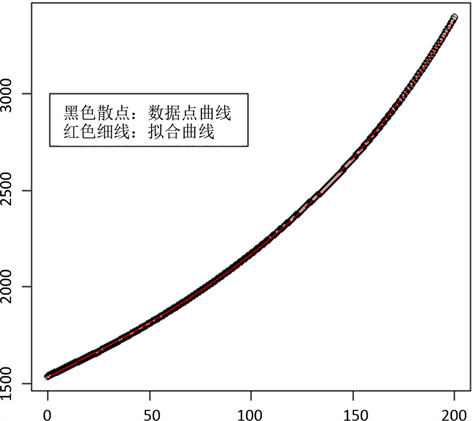Figure 1. Volume of high pressure tube

$V=L\pi {R}^{2}$ (16)

2) 流入高压油管的燃油流量 ${Q}_{1}$

${Q}_{1}=C{A}_{1}\sqrt{\frac{2|{P}_{2}-{P}_{1}|}{\rho }}=0.85×\pi \frac{{1.4}^{2}}{4}\sqrt{\frac{2\cdot |160-{P}_{前}|}{{\rho }_{前}}}$ (17)

3) 高压油管流出的燃油流量 ${Q}_{2}$

${Q}_{2}=\left\{\begin{array}{l}0\text{ },\text{ }\text{ }\text{ }\text{ }\text{ }\text{ }\text{ }\text{ }\text{ }\text{ }\text{ }\text{ }\text{ }\text{ }\text{ }\text{ }\text{ }\text{ }\text{ }\text{ }\text{ }\text{ }\text{ }\text{ }\text{ }\text{ }\text{ }\text{ }\text{ }\text{ }\text{ }\text{ }\text{ }\text{ }\text{ }\text{ }\text{ }\text{ }\text{ }\text{ }\text{ }\text{ }\text{ }\text{ }\text{ }\text{ }\text{ }\text{ }\text{ }\text{ }\text{ }\text{ }\text{ }\text{ }\text{ }\text{ }\text{ }\text{ }\text{ }\text{ }\text{ }\text{ }\text{ }\text{ }\text{ }\text{ }\text{ }\text{ }\text{ }\text{ }\text{ }\text{ }\text{ }\text{ }\text{ }\text{ }\text{ }\text{ }\text{ }\text{ }\text{ }\text{ }\text{ }\text{ }\text{ }\text{ }\text{ }\text{ }\text{ }rem>2.4\text{\hspace{0.17em}}\text{ }\text{or}\text{\hspace{0.17em}}\text{ }rem=0\\ -100×rem+245,\text{ }\text{ }\text{ }\text{ }\text{ }\text{ }\text{ }\text{ }\text{ }\text{ }\text{ }\text{ }\text{ }\text{ }\text{ }\text{ }\text{ }\text{ }\text{ }\text{ }\text{ }\text{ }\text{ }\text{ }\text{ }\text{ }\text{ }\text{ }\text{ }\text{ }\text{ }\text{ }\text{ }\text{ }\text{ }\text{ }2.2 (18)Table 2. Opening time of one-way valveFigure 2. Schematic diagram of pressure variation with time for different opening time of one-way valve

5.2. 模型二：高压油泵运作模型

5.2.1. 模型的准备

1) 凸轮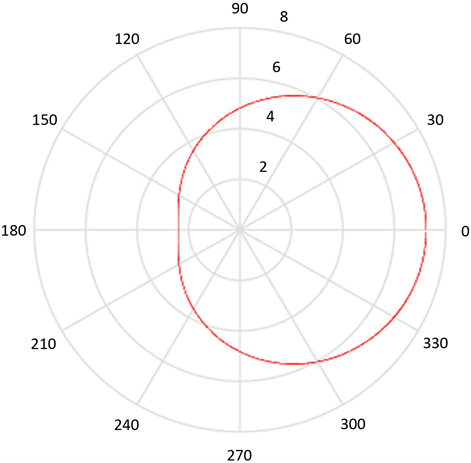Figure 3. Edge Curve of Cam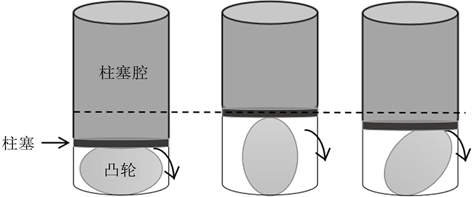Figure 4. Simple schematic diagram of high pressure oil pump

2) 针阀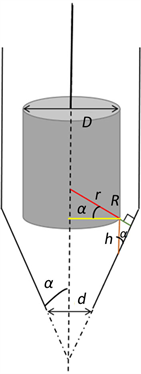Figure 5. Operation diagram of needle valve

$\left\{\begin{array}{l}{L}_{R}=\frac{D}{2}\cdot \frac{1}{\mathrm{cos}\alpha }+h\cdot \mathrm{sin}\alpha \\ {L}_{r}=\frac{D}{2}\cdot \frac{1}{\mathrm{cos}\alpha }\\ {R}_{R}=\mathrm{cos}\alpha \cdot {L}_{R}\\ {R}_{r}=\frac{D}{2}\end{array}$ (19)

$\begin{array}{c}S=\pi {R}_{R}{L}_{R}-\pi {R}_{r}{L}_{r}\\ =\pi \mathrm{cos}\alpha \cdot {\left(\frac{D}{2}\cdot \frac{1}{\mathrm{cos}\alpha }+h\mathrm{sin}\alpha \right)}^{2}-\pi \frac{{D}^{2}}{4}\cdot \frac{1}{\mathrm{cos}\alpha }\end{array}$ (20)

5.2.2. 模型的建立

$\frac{\text{d}{P}_{c}}{\text{d}t}=\frac{E}{{V}_{c}}\left({Q}_{{c}_{1}}-{Q}_{{c}_{2}}\right)$ (21)

1) 压入高压油泵的燃油流量 ${Q}_{{c}_{1}}$

${Q}_{{c}_{1}}=\pi \cdot \frac{{d}_{z}^{2}}{4}\cdot \frac{\text{d}h}{\text{d}t}$ (22)

2) 流入高压油管的燃油流量 ${Q}_{{c}_{2}}$

${Q}_{2}=CA\sqrt{\frac{2\Delta P}{\rho }}=CA\sqrt{\frac{2|{P}_{c}-{P}_{m}|}{{\rho }_{c}}}$ (23)

$\left\{\begin{array}{l}\frac{\text{d}{P}_{c}}{\text{d}t}=\frac{E}{{V}_{c}}\left({Q}_{{c}_{1}}-{Q}_{{c}_{2}}\right)\\ \frac{\text{d}{P}_{m}}{\text{d}t}=\frac{E}{{V}_{m}}\left({Q}_{{m}_{1}}-{Q}_{{m}_{2}}\right)\\ {Q}_{{c}_{1}}=\pi \cdot \frac{{d}_{z}^{2}}{4}\cdot \frac{\text{d}h\left(\omega ,t\right)}{\text{d}t}\\ {Q}_{{c}_{2}}=C{A}_{c}\sqrt{\frac{2|{P}_{c}-{P}_{m}|}{{\rho }_{c}}}\\ {Q}_{{m}_{1}}={Q}_{{c}_{2}}\\ {Q}_{{m}_{2}}=C{A}_{m}\sqrt{\frac{2|{P}_{m}-{P}_{out}|}{{\rho }_{m}}}\end{array}$ (24)

5.2.3. 问题二：确定凸轮的角速度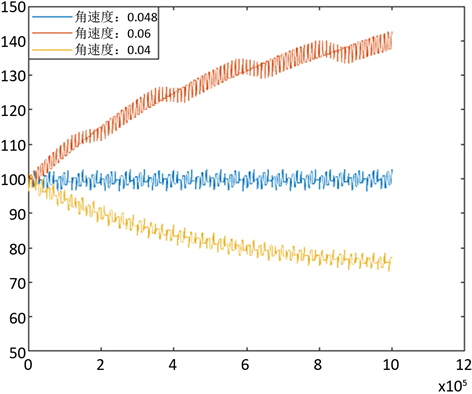Figure 6. Different cam angular velocity pressure changes with time

5.3. 模型三：双喷口高压燃油喷射系统模型

5.3.1. 问题三：增加一个喷油嘴后，喷油和供油策略的调整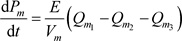(25)

1) 双喷油嘴同时运作Figure 7. Image of pressure in the tubing changing with time when two injectors work together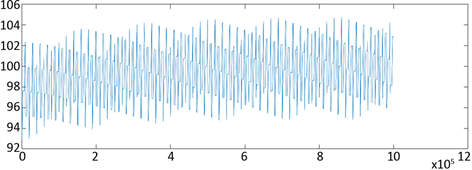Figure 8. When $\omega =0.096\text{\hspace{0.17em}}\text{rad}/\text{ms}$ Image of pressure in the tube as a function of time

${\sigma }^{2}={\left({P}_{{m}_{i}}-{P}_{s}\right)}^{2},i=0,1,\cdots ,100000$ (26)等于0.096 rad/ms且两个喷油嘴同时运作时，其方差为5.6896 × 106

2) 两个喷油嘴邻接运作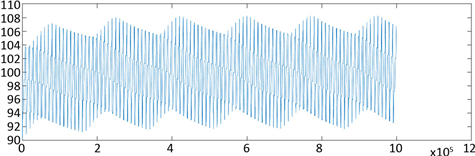Figure 9. Image of pressure change in time two injector nozzles adjacency operating in the oil pipe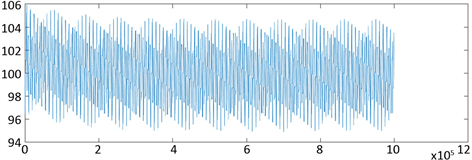Figure 10. Two injectors evenly operate the pressure inside the tubing as a function of time

5.3.2. 问题三：双喷口带单向减压阀的高压燃油喷射系统

$\frac{\text{d}{P}_{m}}{\text{d}t}=\frac{E}{{V}_{m}}\left({Q}_{{m}_{1}}-{Q}_{{m}_{2}}-{Q}_{{m}_{3}}-{Q}_{{m}_{4}}\right)$ (27)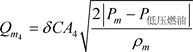(28)

1) 方案一：由时间t决定是否开启单向减压阀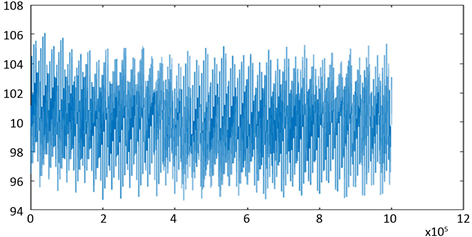Figure 11. When scheme 1 the image of the pressure in the tube changes with time

2) 方案二：由高压油管内的压力 ${P}_{m}$ 决定是否开启单向减压阀

${Q}_{{m}_{5}}=\delta C{A}_{5}\sqrt{\frac{2|{P}_{m}-{P}_{低压燃油}|}{{\rho }_{m}}},\text{ }\text{ }\text{ }\text{ }\text{ }\text{ }\text{ }\text{ }\text{ }\delta =\left\{\begin{array}{l}0,\text{ }\text{ }\text{ }\text{\hspace{0.17em}}{P}_{m}-{P}_{低压燃油}<{P}_{s}\\ 1,\text{ }\text{\hspace{0.17em}}\text{ }\text{ }\text{ }{P}_{m}-{P}_{低压燃油}\ge {P}_{s}\end{array}$ (29)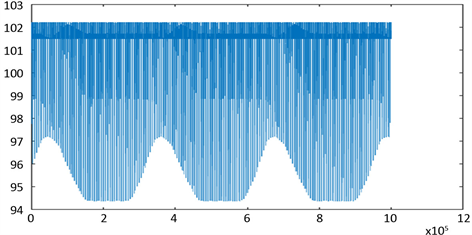Figure 12. When scheme 2 the pressure inside the tube changes with time

6. 模型的评价与改进

6.1. 模型的优点

1) 本文在模型的建立过程中，用到了密度方程、燃油流动的方程以及系统零件的运动方程等，为仿真提供了理论依据。

2) 本文通过对系统结构和工作原理的分析，清楚系统的整个物理过程，建立了系统的详细数学模型，在此基础上，利用MATLAB编写计算仿真的模型。

6.2. 模型的缺点

1) 本文在利用MATLAB模拟仿真时，对高压油泵、高压油管、喷油器等仿真模型都做了一些简化，会导致仿真结果有所误差，同时模拟仿真时内部也会出现系统的误差。

2) 本文在研究过程中，忽略了燃油发热等造成的系统内部温度变化对整个系统的影响，对模型的计算结果可能会造成一定的影响。

6.3. 模型的改进

 2019年国赛A题[EB/OL]. http://cumcm.cnki.net/cumcm//studentHome/studentHome

 王占永. 基于AMEsim的柴油机高压共轨燃油喷射系统的仿真研究[D]: [硕士学位论文]. 西安: 长安大学, 2017.

 张阳明. 基于HYDSIM的高压共轨喷油器设计的仿真研究[D]: [硕士学位论文]. 南京: 南京航空航天大学, 2009.

 张鹏顺, 陆思聪. 弹性流体动力润滑及其应用[M]. 北京: 高等教育出版社, 1995, 13-15.

 王称心. 柴油机高压共轨燃油喷射性能仿真研究[D]: [硕士学位论文]. 无锡: 江南大学, 2015.

 减压阀的工作原理[EB/OL]. https://wenku.baidu.com/view/ae2dfa75dc36a32d7375a417866fb84ae45cc3b7.html, 2019-09-15.

Top